# Preprocessing and event-related activity in combined MEG/EEG data

## Introduction

This tutorial describes how to define epochs-of-interest (trials) from your recorded EEG-MEG-data, and how to apply the different preprocessing steps. This tutorial also shows you how to average your data and compare conditions (standard versus deviant tones).

This tutorial does the preprocessing and segmenting in a single step. If you are interested in how to do preprocessing on your continuous data prior to segmenting it into trials, you can check the Preprocessing - Reading continuous data tutorial.

This tutorial contains the hands-on material of the NatMEG workshop and is complemented by this lecture.

## Background

In FieldTrip the preprocessing of data refers to the reading of the data, segmenting the data around interesting events such as triggers, temporal filtering and (optionally) rereferencing. The ft_preprocessing function takes care of all these steps, i.e., it reads the data and applies the preprocessing options.

There are largely two alternative approaches for preprocessing, which especially differ in the amount of memory required. The first approach is to read all data from the file into memory, apply filters, and subsequently cut the data into interesting segments. The second approach is to first identify the interesting segments, read those segments from the data file and apply the filters to those segments only. The remainder of this tutorial explains the second approach, as that is the most appropriate for large data sets such as the MEG data used in this tutorial. The approach for reading and filtering continuous data and segmenting afterwards is explained in another tutorial.

Preprocessing involves several steps including identifying individual trials from the dataset, filtering and artifact rejections. This tutorial covers how to identify trials using the trigger signal. Defining data segments of interest can be done

• according to a specific trigger channel
• according to your own criteria when you write your own trial function, e.g., for conditional trigger sequences, or by detecting EMG onset

Both depend on ft_definetrial. The output of ft_definetrial is a configuration structure containing the field cfg.trl. This is a matrix representing the relevant parts of the raw datafile which are to be selected for further processing. Each row in the `trl` matrix represents a single epoch-of-interest, and the `trl` matrix has 3 or more columns. The first column defines (in samples) the beginpoint of each epoch with respect to how the data are stored in the raw datafile. The second column defines (in samples) the endpoint of each epoch, and the third column specifies the offset (in samples) of the first sample within each epoch with respect to timepoint 0 within that epoch. The subsequent columns can be used to keep information about each trial.

If you do not specify your own triallfunction, the 4th column will by default contain the trigger value. When you use your own triallfunction, you can add any number of extra columns to the trl matrix. These will be added to the data in the .trialinfo field. This is very handy if you want to add information of e.g., response-buttons, response-times, etc., to each trial. As you will see, we will use this functionality to preprocess both the standard and deviant tones together, and then separating them later for averaging.

## Description of the auditory oddball MEG+EEG dataset

For the EEG-MEG workshop at NatMEG we recorded a dataset of a single subject to allow you to work through all the different steps involved in EEG-MEG analysis: from event-related averaging to frequency analysis, source modeling and statistics.

The experiment that the subject performed is a slight adaptation of the classical oddball experiment. Using the oddball paradigm one can study the well-known EEG component called the mismatch-negativity (MMN). The classical auditory oddball experiment involves the presentation of a continuous series of identical tones at a relatively slow rate, say between one every two seconds to two every second. Every so often, say one out of ten, the tone is slightly different in pitch, duration or loudness. In our version, an oddball occurs after every 3 to 7 standard tones. The interval between each tone is jittered between 700 to 900 ms.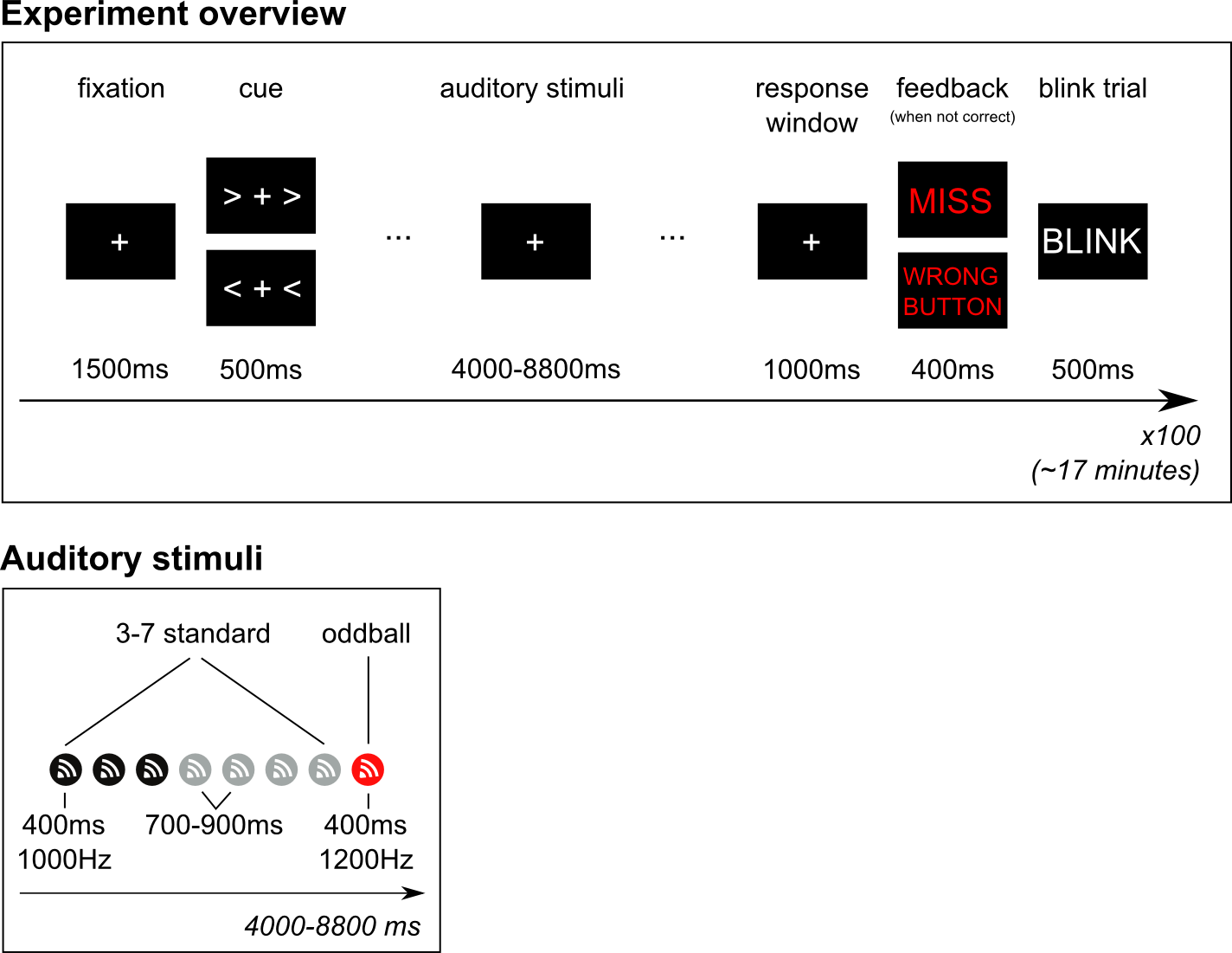### Mismatch negativity

The auditory MMN then occurs as a fronto-central negative EEG potential (relative to the response to the standard tone), with sources in the primary and non-primary auditory cortex and a typical latency of 150-250 ms after the onset of the deviant tone. Not only is the MMN an indicator or auditory functioning, it has also been shown to be under influence of cognitive factors and indicative of cognitive and psychiatric impairments. For a recent and comprehensive overview please see Näätänen et al (2007).

### Cued motor preparation

For the purpose of analyzing oscillatory dynamics we wanted the oddball paradigm to include motor responses, specifically left and right button-presses with the left and right index finger, respectively. The subject was therefor cued to respond with their left or right index finger whenever, and as soon as possible, after a deviant tone occurred. The experiment therefore consistent of series of standard tones + deviant, preceded by a cue (left or right), and followed by a button-press.

### Beta suppression and rebound

It is well known that power in the beta band (15–30 Hz) decreases prior to movement onset but showed a marked sudden increase beginning approximately 300 to 400 ms after termination of EMG activity and lasting for over 500 ms. This post-movement beta rebound (PMBR) is localized to bilateral regions of the pre-central gyrus, but with greater lateralization to the contralateral hemisphere. Contrasting left versus right responses should therefor give us a nice lateralized beta rebound in the pre-central gyrus.

For a recent overview of sensorimotor rhythms, including the beta rebound, please see Cheyne (2013).

Before the recording, the subject performed the experiment in a short training session to get acquainted with the task. Whenever the subject was too late in responding (>2 seconds), or pressed the wrong button, feedback was provided. In the actual experiment the subject was always on time and responded with the correct hand each time. Finally, after each response, a blink trial is presented in which subjects are asked to blink so that they can remain fixated on the fixation cross - without blinking - throughout the period in time in which we are interested in the brain signal.

### Stimuli

The standard tones were 400ms 1000Hz sine-waves, with a short 50ms ramping up- and down to avoid a clicking sound. The oddball tones were identical except for being 1200Hz. The auditory stimuli are presented with an external device that stores uncompressed audio files (.wav) that can then be presented with sub-millisecond precision (in effect instantaneous). Sound was presented through flat-panel sound showers. We measured the duration between the arrival of the triggers (see below) in the data and the arrival of the sound through the air to the ears of the subject. This takes 7.0 ms.

### Triggers

The MEG system records event-triggers in a separate channels, called STI101 and STI102. These channels are recorded simultaneously with the data channels, and at the same sampling rate. The onset (or offset) can therefore be precisely timed with respect to the data. The following trigger codes can be used for the analysis we will be doing during the workshop:

• Onset of standard stimulus: 1
• Onset of oddball stimulus: 2
• Button-press onset of left hand: 256
• Button-press onset of right hand: 4096

### Data

• Data was sampled at 1000Hz.
• 306 channels MEG of which 102 are magnetometers, and 204 are planar gradiometers.
• 128 electrode EEG. The reference was placed on the right mastoid, the ground on the left mastoid. The locations of the electrodes are placed according to the 5% system, which is an extension of the standard 10-20 system for high-density EEG caps. You can find details in Oostenveld and Praamstra, (2001). In addition, the locations of the EEG electrodes was measured in 3D using the Polhemus system and recorded in the data.
• Horizontal EOG(1) electrodes were placed just outside the left and right eye. Vertical EOG(2) were placed above and below the left eye.
• Electrocardiogram (ECG) was recorded as a bipolar recording from the collarbones.
• Electromyography of the lower arm flexors of the left(1) and right(2) arm were recorded.

## Browsing the data prior to preprocessing

Before we start preprocessing our data and calculating event-related fields and potentials, we will first have a look at our data while it unprocessed and not yet cut-up into trials (in FieldTrip parlour: raw-data). To do this, we use ft_databrowser. Note that ft_databrowser is very memory efficient, as it does not read all data in memory - only the part that it displays.

### How can I use the databrowser?

The databrowser can be used to look at your raw or preprocessed data and annotate time periods at which specific events happen. Originally designed to identify sleep spindles, it’s current main purpose is to do quality checks and visual artifact detection.

The databrowser supports three viewmodes: butterfly, vertical or component. In ‘butterfly’ viewmode, all signal traces will be plotted on top of each other, in ‘vertical’ viewmode, the traces will be below each other. The ‘component’ viewmode is to be used for data that is decomposed into independent components, see ft_componentanalysis. Components will be plotted as in the vertical viewmode, but including the coponent topography to the left of the time trace. As an alternative to these three viewmodes, if you provide a cfg.layout, then the function will try to plot your data according to the sensor positions specified in the layout.

When the databrowser opens, you will see buttons to navigate along the bottom of the screen and buttons for artifact annotation to the right. Note that also artifacts that were marked with the automatic artifact detection methods will be displayed here, see the automatic artifact rejection tutorial. You can click on one of the artifact types, drag over a timewindow to select the start and the end of the artifact and then double click into the selected area to mark this artifact. To remove such an artifact, simply repeat the same procedure.

The databrowser will not change your data in any way. If you specify a cfg as output, it will just store your selected or de-selected artifacts in your cfg.

### Visualization combined data

Since we have a dataset that contains both MEG and EEG data, we will browse through the dataset looking at different channel subsets at a time. We will first look at the MEG data. As you know, the Neuromag/Elekta/Megin MEG data has two types of channels; magnetometers and planar gradiometers, we will look at them separately as well. If you are not familiar yet with the difference between different MEG sensor designs, take a look here in this video.

The MEG dataset that we use in this tutorial is available as oddball1_mc_downsampled.fif from our download server.

``````cfg = [];
cfg.dataset    = 'oddball1_mc_downsampled.fif';
cfg.continuous = 'yes';
cfg.channel    = 'MEG*1';
cfg.viewmode   = 'vertical';
cfg.blocksize  = 1; % Length of data to display, in seconds

ft_databrowser(cfg);

set(gcf, 'Position',[1 1 1200 800])
print -dpng natmeg_databrowser1.png
``````

If your recorded data is continuous, specify cfg.continuous = ‘yes’, if you have recorded your data in trials, specify cfg.continuous = ‘no’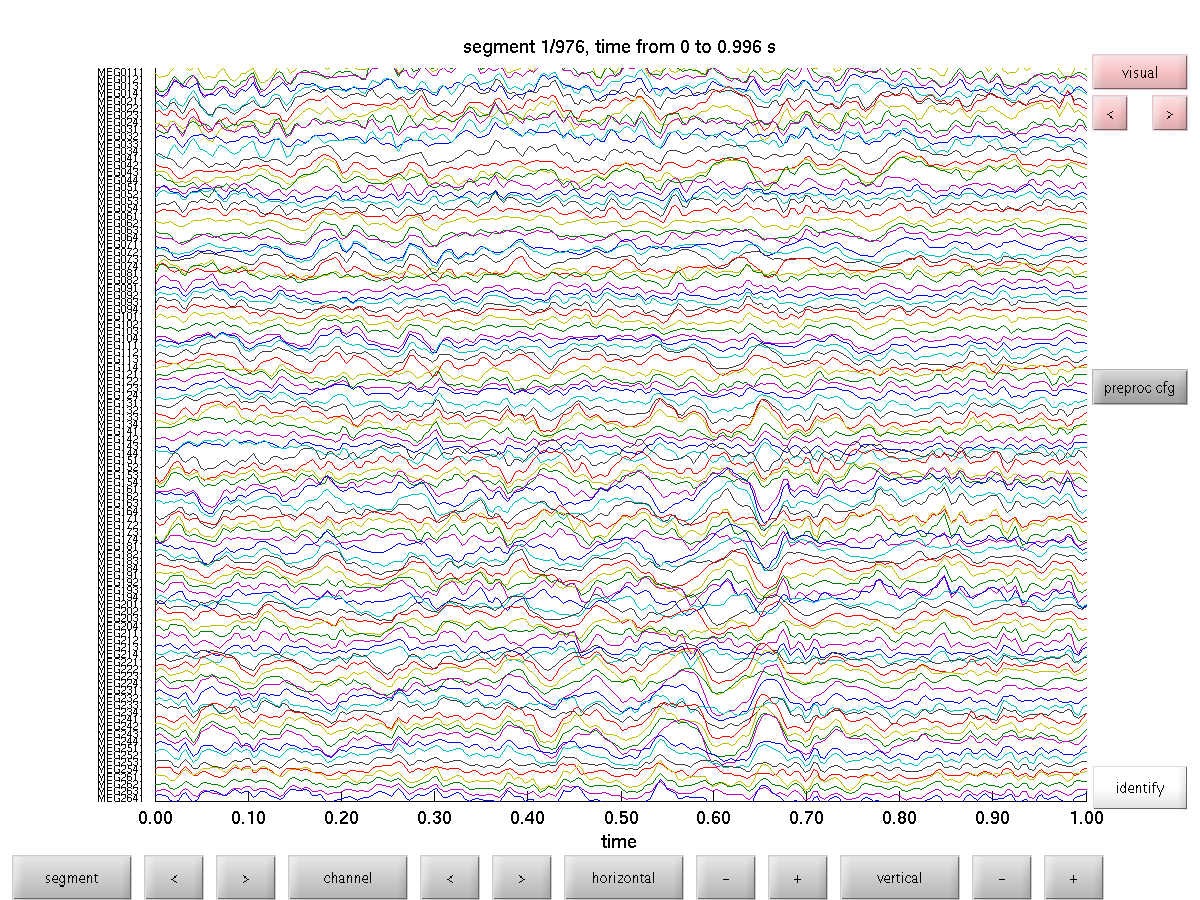Figure: Raw plot from magnetometers using ft_databrowser

Try to get a feel of your data by browsing through the data. Do you see any obvious artifacts?

Now we will have a look at the gradiometer

``````cfg = [];
cfg.dataset = 'oddball1_mc_downsampled.fif';
cfg.channel = {'MEG*2', 'MEG*3'};
cfg.viewmode = 'vertical';
cfg.blocksize = 1;                             % Length of data to display, in seconds

ft_databrowser(cfg);
``````

Finally, we will look at the EEG channel

``````cfg = [];
cfg.dataset = 'oddball1_mc_downsampled.fif';
cfg.channel = 'EEG';
cfg.viewmode = 'vertical';
cfg.blocksize = 1;                             % Length of data to display, in seconds
cfg.preproc.demean = 'yes';                    % Demean the data before display
cfg.ylim = [-4e-6 4e-6];

ft_databrowser(cfg);

set(gcf, 'Position',[1 1 1200 800])
print -dpng natmeg_databrowser2.png
``````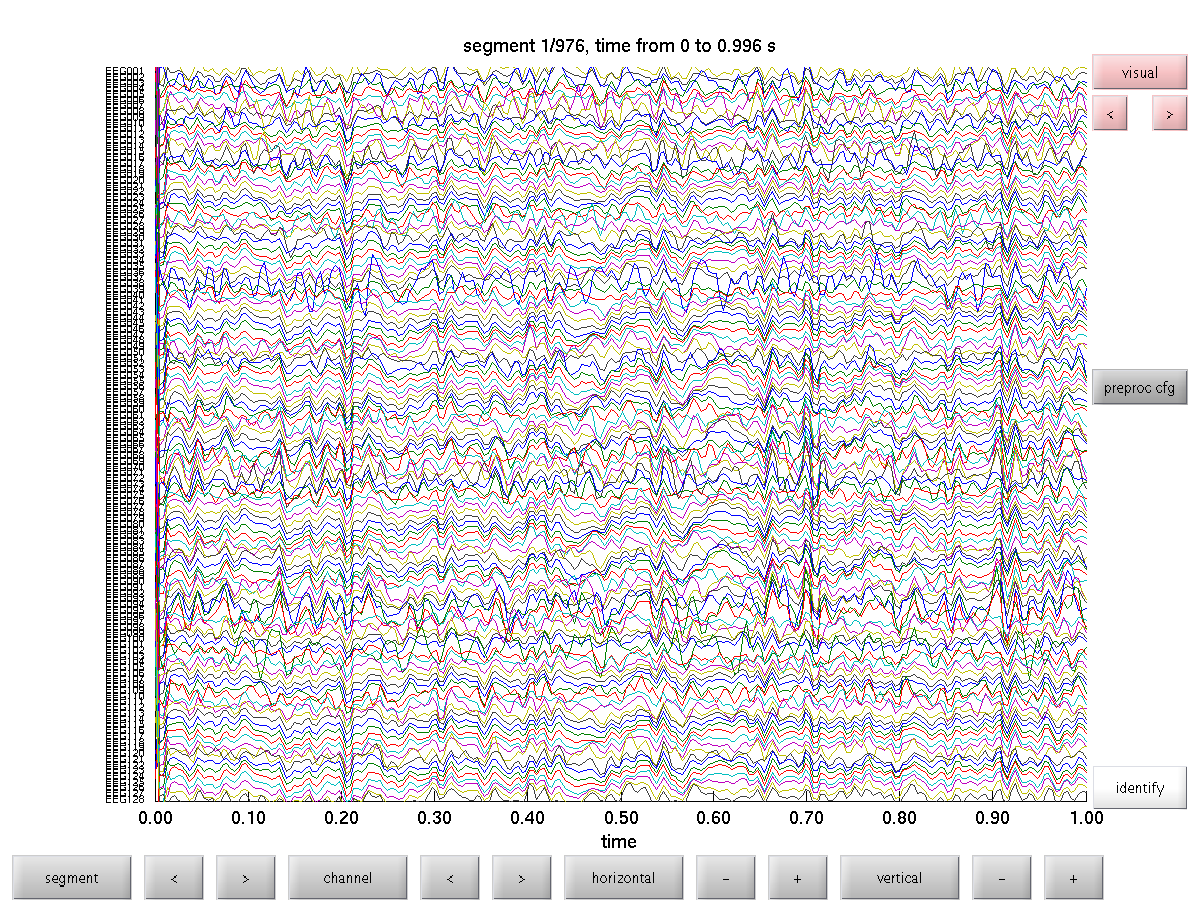Figure: Raw plot from EEG channels using ft_databrowser

At first glance, can you see any differences between the MEG and EEG data or artifacts?

## Preprocessing and averaging MEG

### Procedure

The following steps are taken in the MEG section of the tutorial: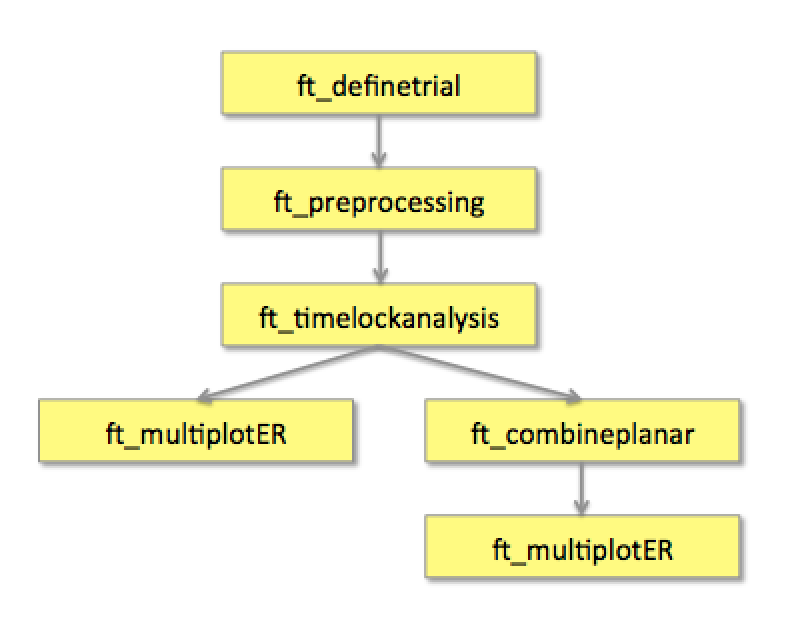Figure: A schematic overview of the steps in averaging of event-related fields

### Reading and preprocessing the interesting trials

Using the FieldTrip function ft_definetrial you can define the segments of data that will be read in for preprocessing. Trials are defined by their begin and end-sample in the data file and each trial has an offset that defines where the relative t=0 point (usually the moment of stimulus onset, i.e. on the stimulus-trigger) is for that trial.

The MEG dataset that we use in this tutorial is available as oddball1_mc_downsampled.fif from our download server. Furthermore, you should download and save the custom trial function trialfun_oddball_stimlocked.m to a directory that is on your MATLAB path.

We will now do the trial definition for both the standard and deviant trial

``````cfg = [];
cfg.dataset = 'oddball1_mc_downsampled.fif';

cfg.trialdef.prestim        = 1;
cfg.trialdef.poststim       = 1;
cfg.trialdef.std_triggers   = 1;
cfg.trialdef.stim_triggers  = [1 2]; % 1 for standard, 2 for deviant
cfg.trialdef.odd_triggers   = 2;
cfg.trialdef.rsp_triggers   = [256 4096];
cfg.trialfun                = 'trialfun_oddball_stimlocked';
cfg                         = ft_definetrial(cfg);
``````

This results in a cfg.trl in which the beginning, the trigger offset and the end of each trial relative to the beginning of the raw data is defined. In addition, we’ve added an extra column in the .trl that describing whether the trial consist of a normal tone (1) or deviant (2). We will use this later to separately average these conditions. You can find more details about the trialinfo field in the FAQ: Is it possible to keep track of trial-specific information in my FieldTrip analysis pipeline? and Making your own trialfun for conditional trial definition.

The output of ft_definetrial is an updated cfg structure that can be used for ft_preprocessing, which uses the information about the start-sample, end-sample and offset to cut it up in separate trials and to align the segments to each other.

``````cfg.continuous              = 'yes';
cfg.hpfilter                = 'no';
cfg.detrend                 = 'no';
cfg.continuous              = 'yes';
cfg.demean                  = 'yes';
cfg.dftfilter               = 'yes';
cfg.dftfreq                 = [50 100];
cfg.channel                 = 'MEG';

data_MEG                    = ft_preprocessing(cfg);
``````

Save the preprocessed data to dis

``````save data_MEG data_MEG -v7.3
``````

The output of ft_preprocessing is the structure data_MEG which has the following field

``````data_MEG =

hdr: [1x1 struct]
label: {306x1 cell}    % Channel names
time: {1x600 cell}    % Array of time points considered
trial: {1x600 cell}    % The data of individual trials
fsample: 250             % Sampling rate
sampleinfo: [600x2 double]  % Samples in the original data corresponding to trials
trialinfo: [600x3 double]  % Trial bookkeeping
elec: [1x1 struct]    % Electrode positions etc
cfg: [1x1 struct]    % Settings used in computing this time-locked analysis
``````

The most important fields are data_MEG.trial containing the individual trials and data_MEG.time containing the time vector for each trial. To visualize the single trial data (trial 1) on one channel (channel 130) do the following:

``````plot(data_MEG.time{1}, data_MEG.trial{1}(130,:))
print -dpng natmeg_preproc1.png
``````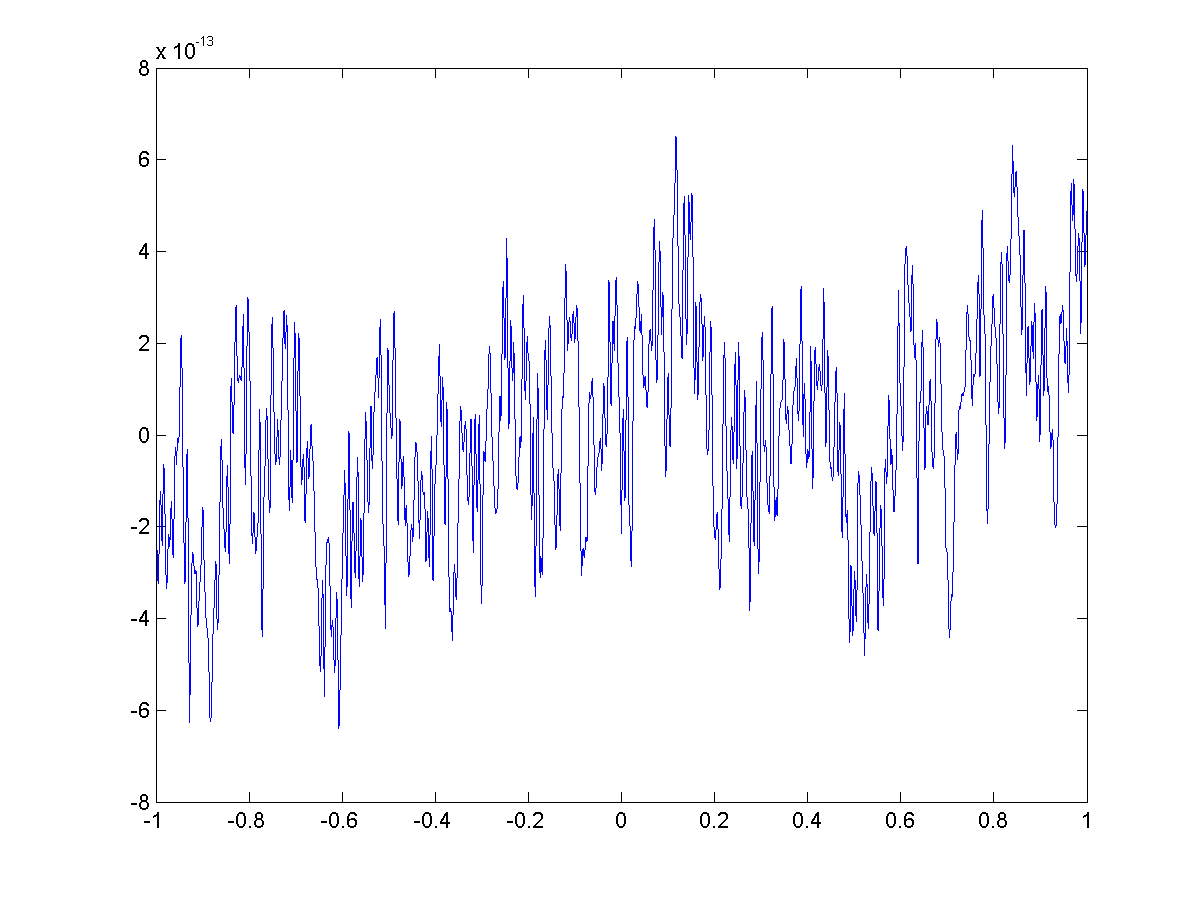Figure: A plot of a single trial of one channel

This demonstrate how to extract trials from a dataset based on trigger information. Note that some of these trials will be contaminated with various artifact such as eye blinks or MEG sensor jumps. The way we deal with artifacts is described in general in another tutorial and visual artifact rejection is described in the Visual artifact rejection tutorial. For efficiency in this hands-on tutorial, we will use a semi-automatic way of rejecting trials and channels containing artifacts using a summary view of all trials and channels transformed into z-scores. This allows you to get a quick overview of your data and enables you to easily detect and remove outliers.

``````% separately for magnetometers
cfg               = [];
cfg.metric        = 'zvalue';
cfg.layout        = 'neuromag306all.lay';
cfg.channel       = 'MEG*1';
cfg.keepchannel   = 'yes';  % This keeps those channels that are not displayed in the data
data_MEG_clean    = ft_rejectvisual(cfg,data_MEG);

cfg.channel = {'MEG*2','MEG*3'};
data_MEG_clean    = ft_rejectvisual(cfg,data_MEG_clean);
``````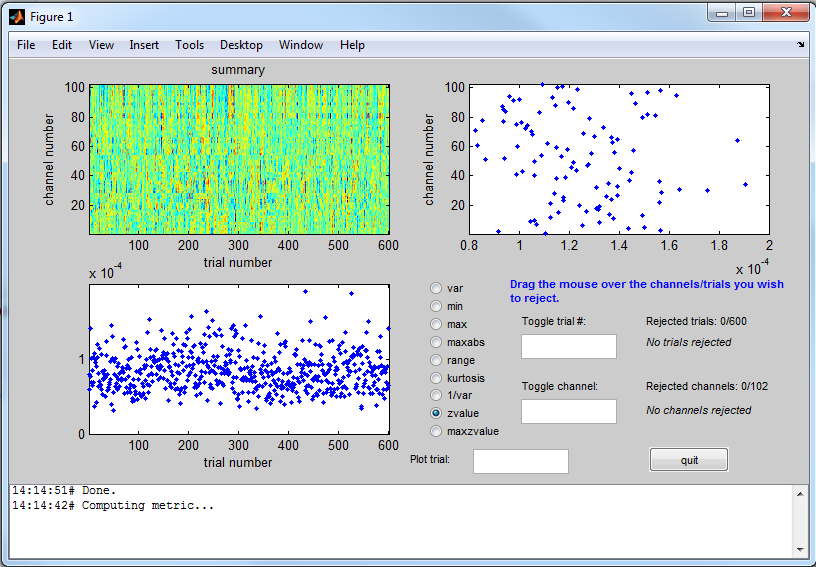Figure: An overview of the data using ft_rejectvisual

Use the mouse to click and drag a selection box over the trials you wish to reject. You can see the trials that were marked for rejection on the right. If you wish to unmark a trial for rejection, type the number of the trial in the ‘toggle trial’ box and press enter. You can change from zvalue to another metrics to detect outliers.

Please be aware that while cfg.keepchannel=’yes’ is specified, you can disable channels in the display but those will not be removed from the data.

After we have rejected trials with artifacts we will save our dat

``````save data_MEG_clean data_MEG_clean -v7.3
``````

We analyze EEG or MEG signals to investigate the modulation of the measured brain signals with respect to a certain event/stimulus. However, due to intrinsic and extrinsic noise in the signals - which in single trials is often higher than the signal evoked by the brain - it is typically required to average data from several trials to increase the signal-to-noise ratio (SNR). One approach is to repeat a given event/stimulus in your experiment and average the corresponding EEG or MEG signals. The assumption that we rely on here is that the noise is independent of the events and thus reduced when averaging, while the effect of interest is present in each trial and time-locked to the event. The approach results in event-related Potentials (ERPs) or event-related Fields (ERFs) for EEG and MEG, respectively.

#### Timelockanalysis

The function ft_timelockanalysis makes an average over all the trials in a segmented raw data structure. It requires preprocessed data, i.e. what we just did.

``````load data_MEG_clean
``````

We will first apply some additional filters for visualization purposes using ft_preprocessing.

``````cfg = [];
cfg.lpfilter        = 'yes';
cfg.lpfreq          = 25;
cfg.demean          = 'yes';
cfg.baselinewindow  = [-0.5 0];
data_MEG_filt       = ft_preprocessing(cfg,data_MEG_clean);
``````

The trials belonging to one condition will now be averaged with the onset of the stimulus time aligned to the zero-time point (the onset of the last word in the sentence). This is done with the function ft_timelockanalysis. The input to this procedure is the data_EEG structure generated by ft_preprocessing.

We will use cfg.trials to specify which trials should go into the average. We will use this to split the data into the oddball and standard trials. The cfg.trials field is simply a vector with the trial indices of the trials we want to average. Since the trigger core is in data.trialinfo, we can use that to select the trials of interest.

``````cfg = [];
cfg.trials          = find(data_MEG_filt.trialinfo(:,1) == 1);
ERF_standard        = ft_timelockanalysis(cfg,data_MEG_filt);

cfg.trials          = find(data_MEG_filt.trialinfo(:,1) == 2);
ERF_oddball         = ft_timelockanalysis(cfg,data_MEG_filt);
``````

We will also calculate the difference between both conditions using ft_math.

``````cfg = [];
cfg.operation = 'subtract';
cfg.parameter = 'avg';

ERF_diff = ft_math(cfg, ERF_oddball, ERF_standard);
``````

The output is the data structure ERF_standard with the following field

``````ERF_standard =

avg: [306x501 double]
var: [306x501 double]
time: [1x501 double]
dof: [306x501 double]
label: {306x1 cell}
dimord: 'chan_time'
elec: [1x1 struct]
cfg: [1x1 struct]
``````

The most important field is ERF_standard.avg, containing the average over all trials for each sensor.

#### Plotting the results using the magnetometers

Using the plot functions ft_multiplotER, ft_singleplotER and ft_topoplotER you can make plots of the average. You can find information about plotting also in the Plotting data at the channel and source level tutorial.

Use ft_multiplotER to plot all sensors in one figure:

``````cfg = [];
cfg.fontsize = 6;
cfg.layout = 'neuromag306mag.lay';
cfg.ylim = [-2.5e-13 2.5e-13];
cfg.xlim = [-0.2 0.6];

figure
ft_multiplotER(cfg, ERF_standard, ERF_oddball, ERF_diff );
legend({'Standard';'Oddball';'Difference'});

set(gcf,'Position',[1 1 1239 945]);
print -dpng natmeg_erf1.png
``````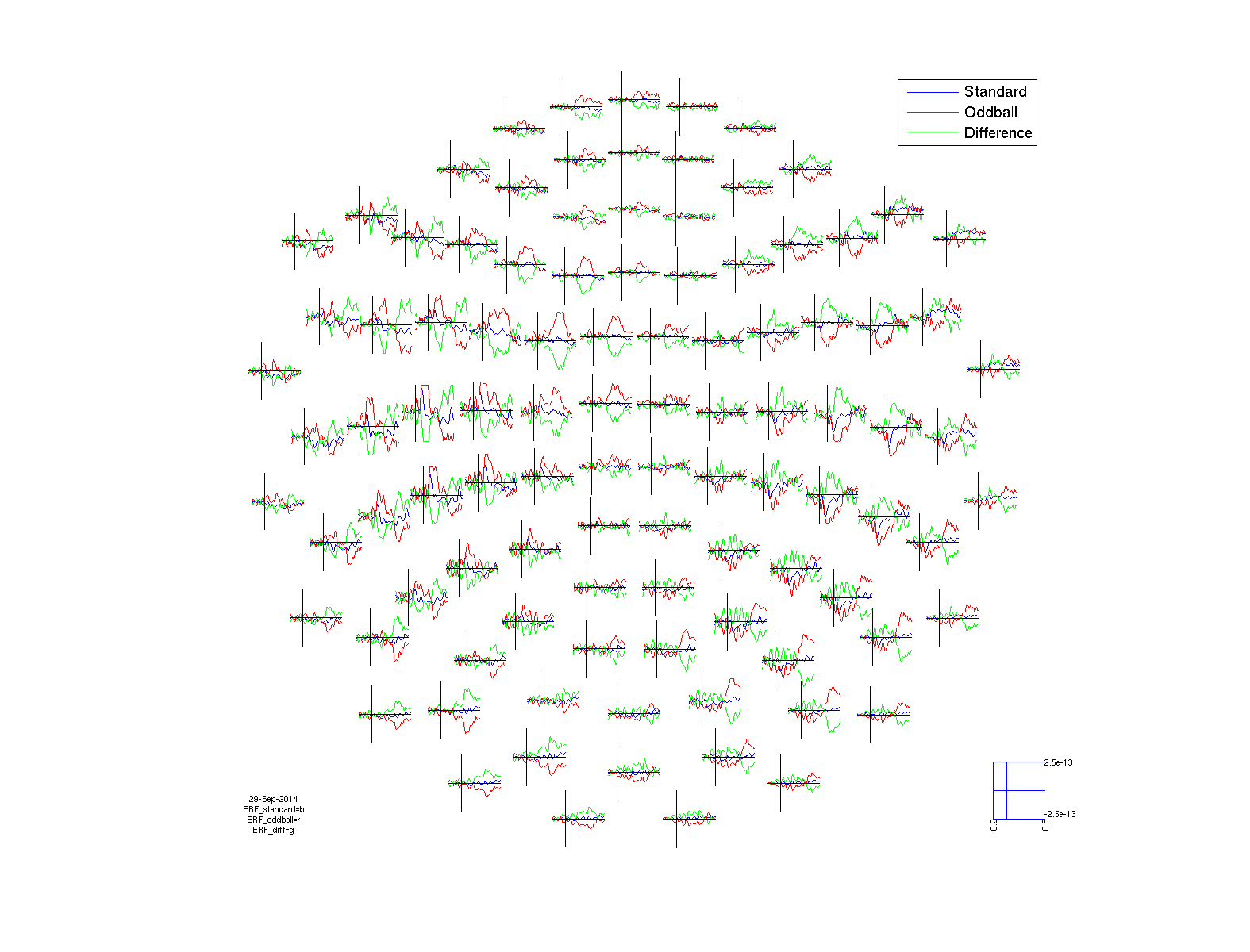Figure: A plot of the average of all conditions for all channels plotted using ft_multiplotER

This plots the event-related fields for all sensors arranged topographically according to their position in the helmet. You can use the zoom button (magnifying glass) to enlarge parts of the figure.

To plot one sensor data use ft_singleplotER and specify the name of the channel you are interested in, for instance ‘MEG0211

``````cfg = [];
cfg.fontsize = 6;
cfg.layout   = 'neuromag306mag.lay';
cfg.xlim     = [-0.2 0.6];
cfg.ylim     = [-3e-13 3e-13];
cfg.channel  = 'MEG0211';

figure;
ft_singleplotER(cfg, ERF_standard, ERF_oddball, ERF_diff);
legend({'Standard';'Oddball';'Difference'});

print -dpng natmeg_erf2.png
``````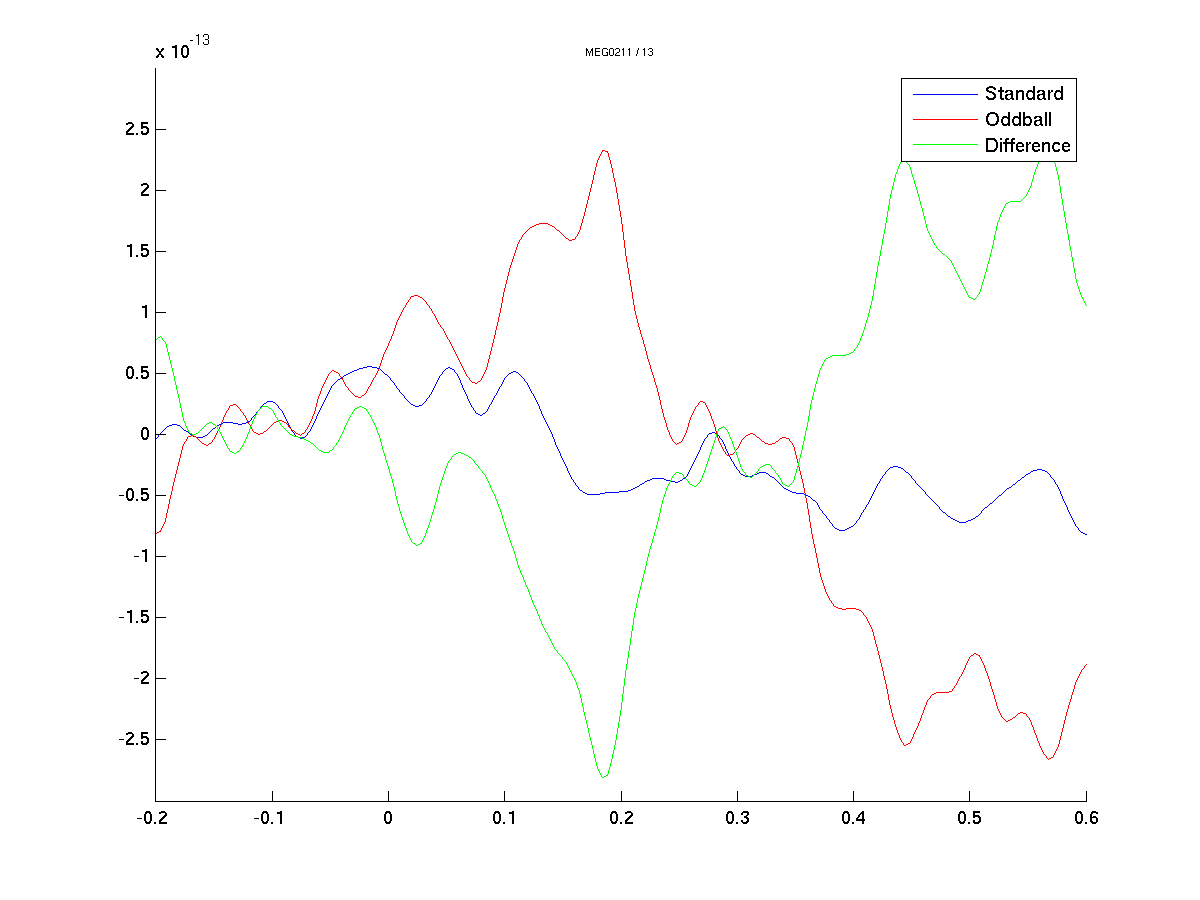Figure: A plot of the average of all conditions for channel MEG0211 plotted using ft_singleplotER

To plot the topographic distribution of the data averaged over the time interval from 0.08 to 0.15 seconds use to following command

``````cfg                 = [];
cfg.layout          = 'neuromag306mag.lay'; % name will change
cfg.zlim            = [-3e-13 3e-13];
cfg.xlim            = [0.08 0.15];
cfg.style           = 'straight';
cfg.comment         = 'no';
cfg.marker          = 'off';
cfg.colorbar        = 'southoutside';

figure;
subplot(1,3,1);
ft_topoplotER(cfg, ERF_standard);
title('Standard');
axis tight

subplot(1,3,2);
ft_topoplotER(cfg, ERF_oddball);
title('Oddball');
axis tight

subplot(1,3,3);
ft_topoplotER(cfg, ERF_diff);
title('Difference');
axis tight

print -dpng natmeg_erf3.png
``````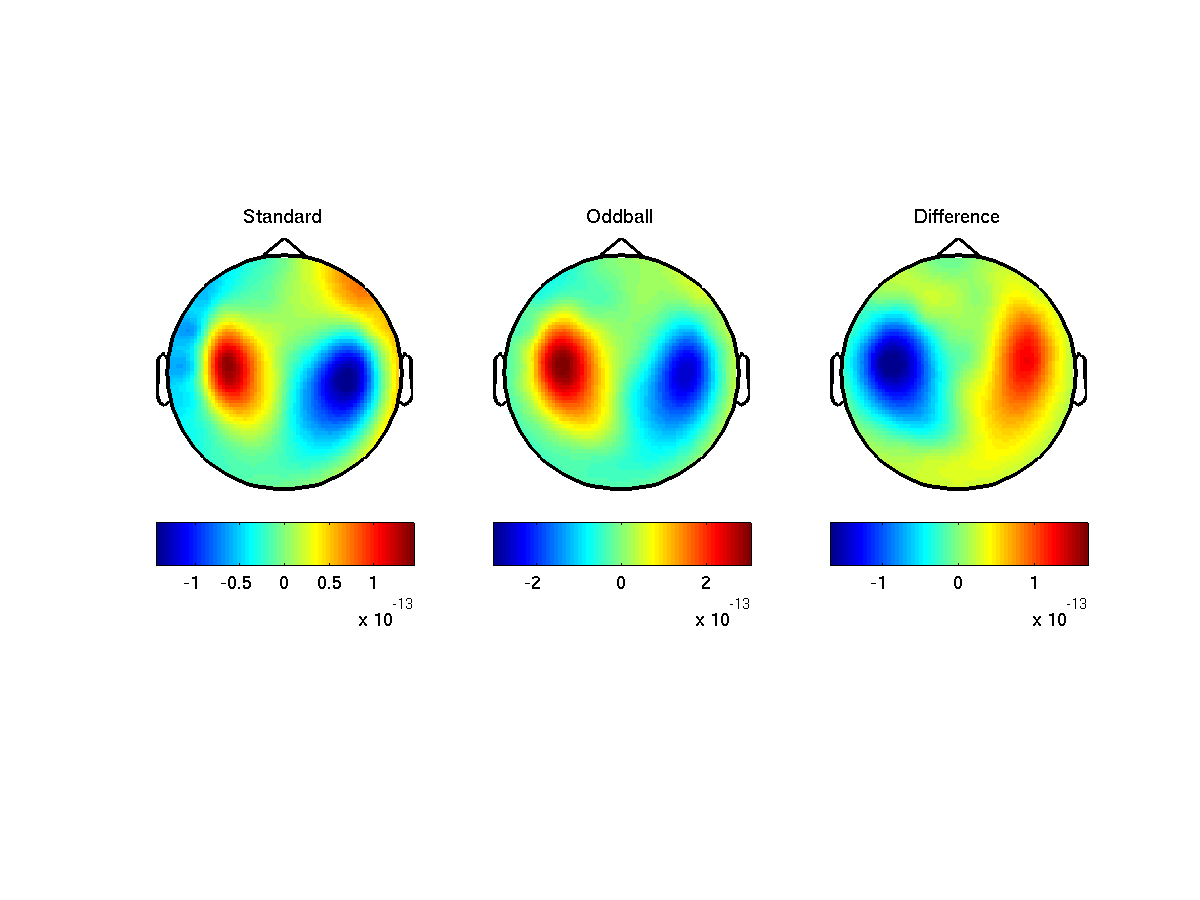Figure: Topoplot of the data averaged between 0.08 and 0.15 seconds using ft_topoplotER

Can you try to explain the topographical distribution in terms of a dipole?

As you could see in the previous section, the magnetometers may give a topographical distribution which can be difficult to interpret. To help with identifying underlying sources we should make use of the other channels in the data. The planar gradiometers are often more easily interpreted, because they are most sensitive right above a source. However, the gradiometers are composed of two (8-shaped) coils at the same location, oriented in two different directions with respect to the surface of the helmet. They can thereby pick up both radial orientations of the magnetic fields. To use them properly for the purpose of plotting, we should therefor combine them first, adding their fields.

``````% Combine planar
cfg = [];
ERF_standard_cmb    = ft_combineplanar(cfg, ERF_standard);
ERF_oddball_cmb     = ft_combineplanar(cfg, ERF_oddball);
ERF_diff_cmb        = ft_combineplanar(cfg, ERF_diff);
``````

#### Plotting the results of planar gradients

We are now going to create the same plots as before, but for the combined planar gradiometers.

Use ft_multiplotER to plot all sensors in one figure:

``````cfg = [];
cfg.fontsize = 6;
cfg.layout   = 'neuromag306cmb.lay';
cfg.ylim     = [0 8e-12];
cfg.xlim     = [-0.2 0.6];

figure;
ft_multiplotER(cfg, ERF_standard_cmb, ERF_oddball_cmb, ERF_diff_cmb);
legend({'Standard', 'Oddball', 'Difference'});

set(gcf,'Position',[1 1 1239 945]);
print -dpng natmeg_erf4.png
``````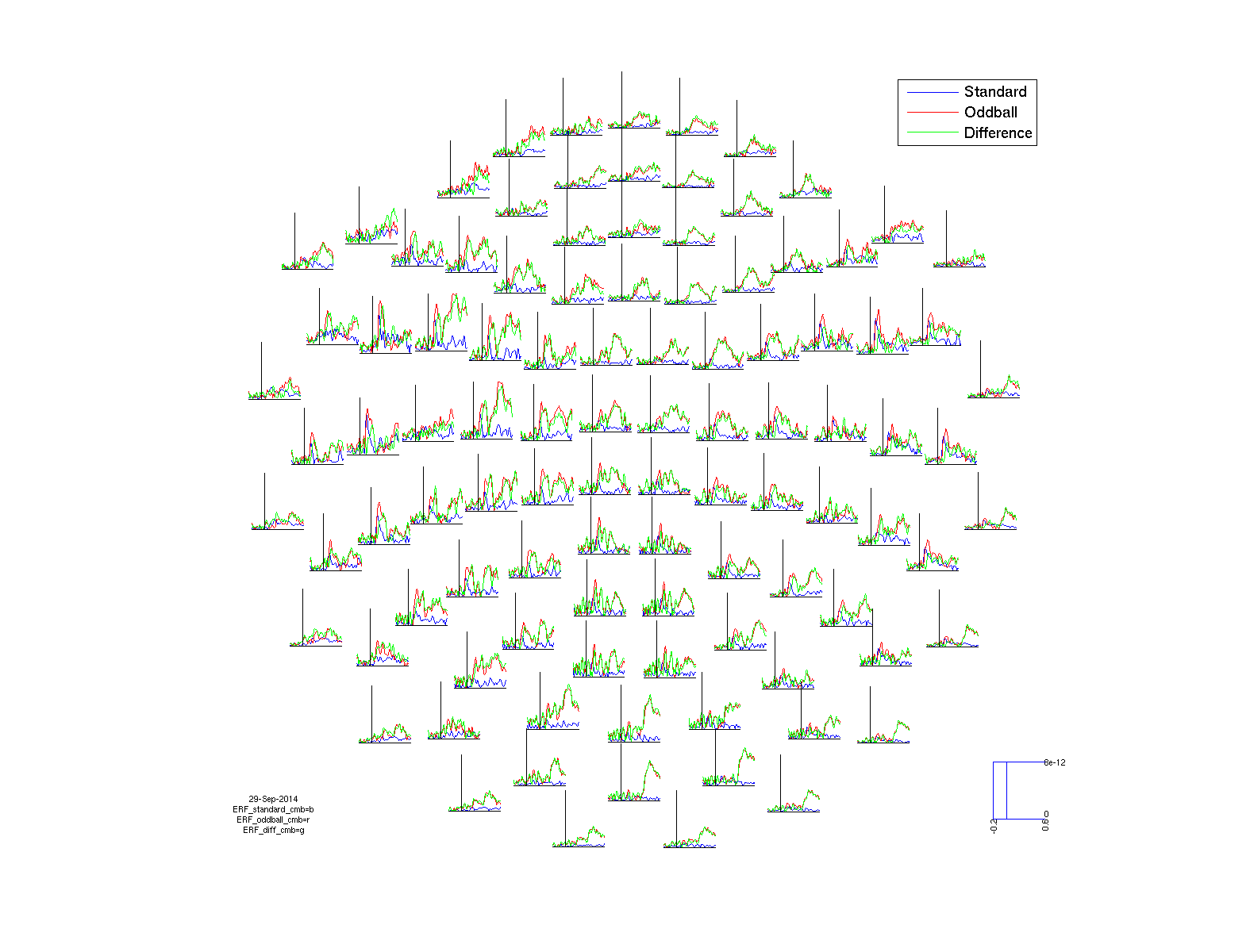Figure: The event-related fields plotted using ft_multiplotER. The event-related fields were calculated using ft_preprocessing followed by ft_timelockanalysis

How does this figure compare to the plot with the magnetometer data? Do you understand why these are different?

We will now zoom in on one combined channel, for instance in the combined channel ‘MEG0222+0223

``````cfg = [];
cfg.showlabels = 'yes';
cfg.fontsize   = 6;
cfg.layout     = 'neuromag306cmb.lay';
cfg.xlim       = [-0.2 0.6];
cfg.ylim       = [0 8e-12];
cfg.channel    = 'MEG0222+0223';

figure;
ft_singleplotER(cfg, ERF_standard_cmb, ERF_oddball_cmb, ERF_diff_cmb);
legend({'Standard', 'Oddball', 'Difference'});

print -dpng natmeg_erf5.png
``````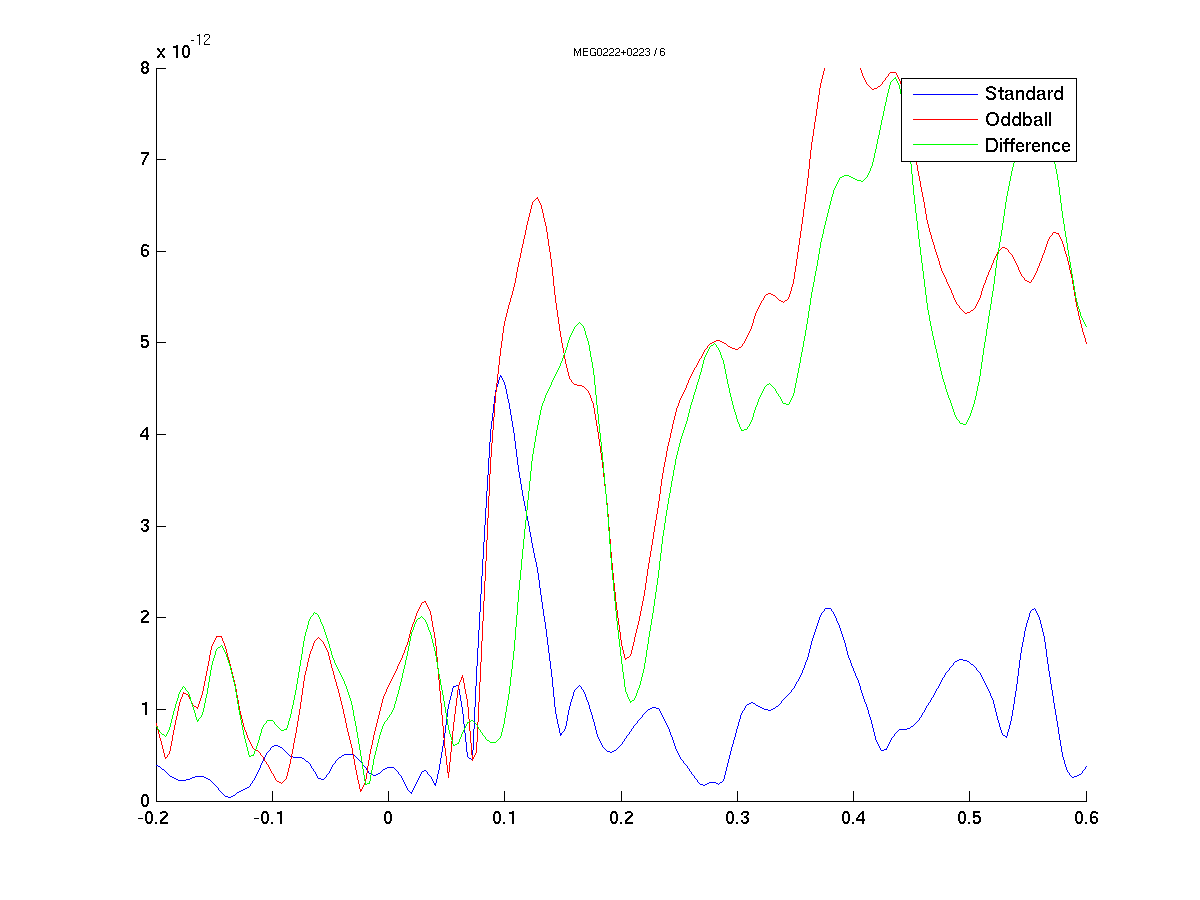Figure: The event-related field plotted using ft_singleplotER. The event-related fields were calculated using ft_preprocessing followed by ft_timelockanalysis

Now we are going to look at the topographical spread of the field by using

``````cfg                 = [];
cfg.layout          = 'neuromag306cmb.lay'; % name will change
cfg.zlim            = 'zeromax';
cfg.xlim            = [0.08 0.15];
cfg.style           = 'straight';
cfg.comment         = 'no';
cfg.marker          = 'off';
cfg.colorbar        = 'southoutside';

figure;
subplot(1,3,1);
ft_topoplotER(cfg, ERF_standard_cmb);
title('Standard');
axis tight

subplot(1,3,2);
ft_topoplotER(cfg, ERF_oddball_cmb);
title('Deviant');
axis tight

subplot(1,3,3);
ft_topoplotER(cfg, ERF_diff_cmb);
title('Difference');
axis tight

print -dpng natmeg_erf6.png
``````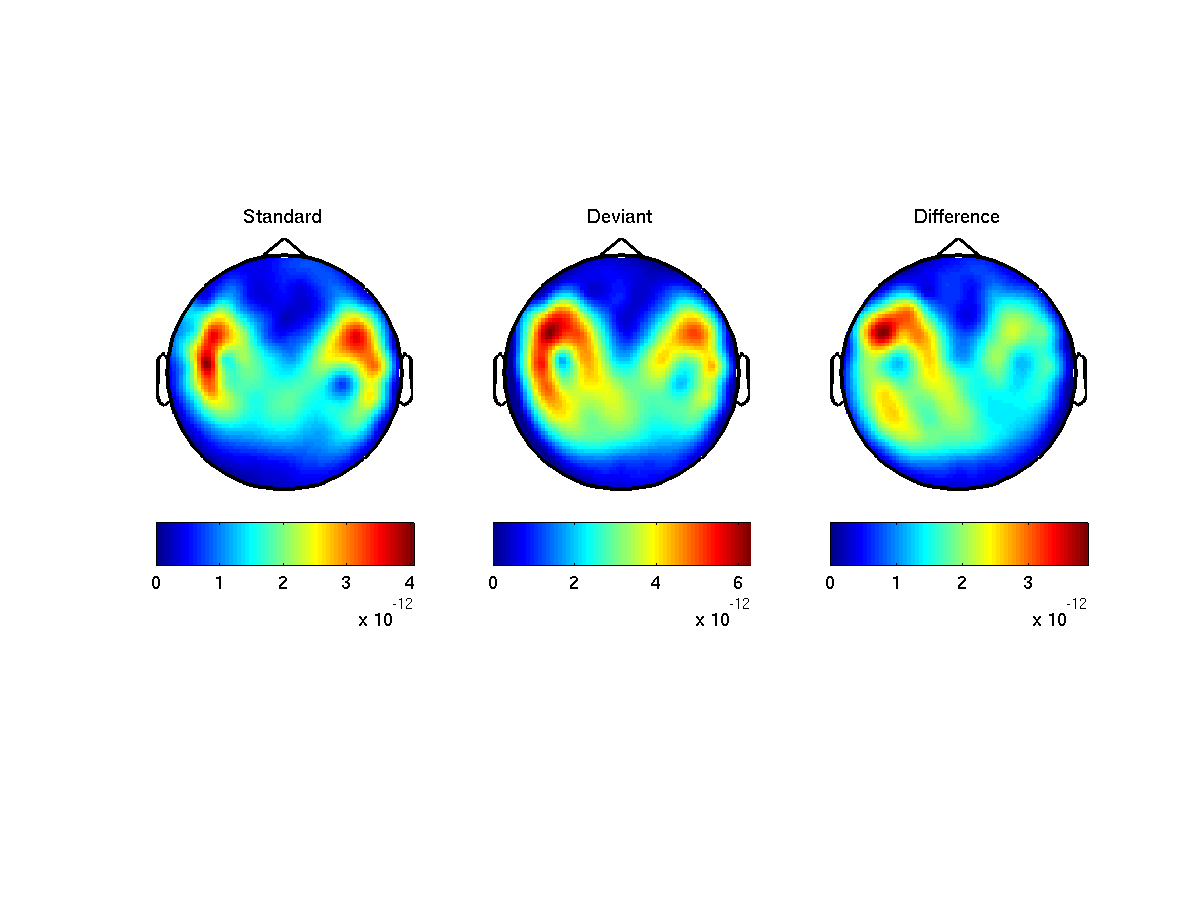Figure: Topographic plot of the event-related fields obtained using ft_topoplotER

Compare this distribution with those resulting from the magnetometers. Do you understand the differences?

Which type of source configuration can explain the topography?

## Preprocessing and averaging EEG

Now that you have looked at the data using the MEG sensors we are going to switch to the EEG sensors. During the following steps we will look back and compare our EEG results to the MEG results. See if you can point out differences and similarities.

### Procedure

The EEG section of this tutorial resembles the MEG section. We will take the following steps: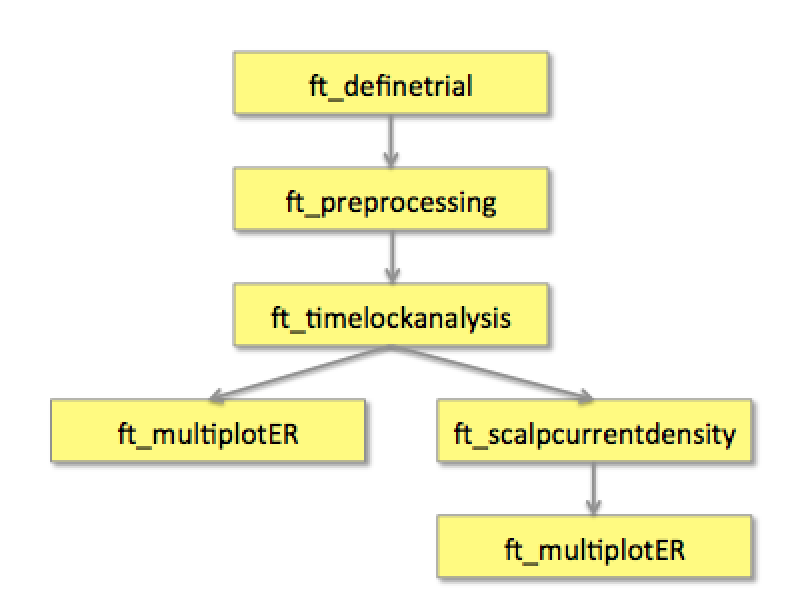A schematic overview of the steps in averaging of event-related potentials

### Reading and preprocessing the interesting trials

We start by repeating the same preprocessing procedure as with the MEG. We start with the trial definition for the standard and oddball trials using ft_definetrial and ft_preprocessing.

``````cfg = [];
cfg.dataset = 'oddball1_mc_downsampled.fif';

cfg.trialdef.prestim        = 1;
cfg.trialdef.poststim       = 1;
cfg.trialdef.std_triggers   = 1;
cfg.trialdef.stim_triggers  = [1 2];
cfg.trialdef.odd_triggers   = 2;
cfg.trialdef.rsp_triggers   = [256 4096];
cfg.trialfun                = 'trialfun_oddball_stimlocked';
cfg                         = ft_definetrial(cfg);

cfg.continuous              = 'yes';
cfg.hpfilter                = 'no';
cfg.detrend                 = 'no';
cfg.continuous              = 'yes';
cfg.demean                  = 'yes';
cfg.dftfilter               = 'yes';
cfg.dftfreq                 = [50 100];
cfg.channel                 = 'EEG';

cfg.reref                   = 'yes'; % recorded with left mastoid
cfg.refchannel              = 'all';

data_EEG                    = ft_preprocessing(cfg);
save data_EEG data_EEG -v7.3
``````

The output of data_EEG is the structure data_EEG which has the following field

``````data_EEG =
hdr: [1x1 struct]
label: {128x1 cell}
time: {1x600 cell}
trial: {1x600 cell}
fsample: 250
sampleinfo: [600x2 double]
trialinfo: [600x3 double]
elec: [1x1 struct]
cfg: [1x1 struct]
``````

As before, we will use ft_rejectartifact to clean the data of bad trials (and perhaps channels).

``````cfg               = [];
cfg.metric        = 'zvalue';
cfg.layout        = 'natmeg_customized_eeg1005.lay';
data_EEG_clean    = ft_rejectvisual(cfg,data_EEG);
``````

The EEG equivalent of the Event-Related Field (ERF) is the Event-Related Potential (ERP). As with the MEG data we will first filter the data using ft_preprocessing before calculating the ERP with ft_timelockanalysis

#### Timelockanalysis

The function ft_timelockanalysis makes an average over all the trials in a segmented raw data structure. It requires preprocessed data, i.e. what we just did.

We will first apply some additional filters for visualization purposes using ft_preprocessing.

``````cfg = [];
cfg.lpfilter        = 'yes';
cfg.lpfreq          = 25;
cfg.demean          = 'yes';
cfg.baselinewindow  = [-0.5 0];
data_EEG_filt       = ft_preprocessing(cfg,data_EEG_clean);
``````

The trials belonging to one condition will now be averaged with the onset of the stimulus time aligned to the zero-time point (the onset of the last word in the sentence). This is done with the function ft_timelockanalysis. The input to this procedure is the data_EEG structure generated by ft_preprocessing. We will use cfg.trials to specify which trials should go into the average and thereby split between the oddball and standard trials.

``````cfg = [];
cfg.trials          = find(data_EEG_filt.trialinfo(:,1) == 1);
ERP_standard        = ft_timelockanalysis(cfg, data_EEG_filt);

cfg.trials          = find(data_EEG_filt.trialinfo(:,1) == 2);
ERP_oddball         = ft_timelockanalysis(cfg, data_EEG_filt);
``````

We will also calculate the difference between both conditions using ft_math.

``````cfg = [];
cfg.operation = 'subtract';
cfg.parameter = 'avg';

ERP_diff = ft_math(cfg, ERP_oddball, ERP_standard);
``````

The output are data structures with the following field

``````ERP_oddball =

avg: [128x2001 double]
var: [128x2001 double]
time: [1x2001 double]
dof: [128x2001 double]
label: {128x1 cell}
dimord: 'chan_time'
elec: [1x1 struct]
cfg: [1x1 struct]
``````

The most important field is ERP_oddball.avg, containing the average over all trials for each sensor.

#### Plotting the results of EEG

Using the plot functions ft_multiplotER, ft_singleplotER and ft_topoplotER you can make plots of the average. You can find information about plotting also in the Plotting data at the channel and source level tutorial.

Use ft_multiplotER to plot all sensors in one figure:

``````cfg          = [];
cfg.fontsize = 6;
cfg.layout   = 'natmeg_customized_eeg1005.lay';
cfg.ylim     = [-3e-6 3e-6];
cfg.xlim     = [-0.2 0.6];

figure;
ft_multiplotER(cfg, ERP_standard, ERP_oddball, ERP_diff);

set(gcf,'Position',[1 1 1239 945]);
print -dpng natmeg_erp1.png
``````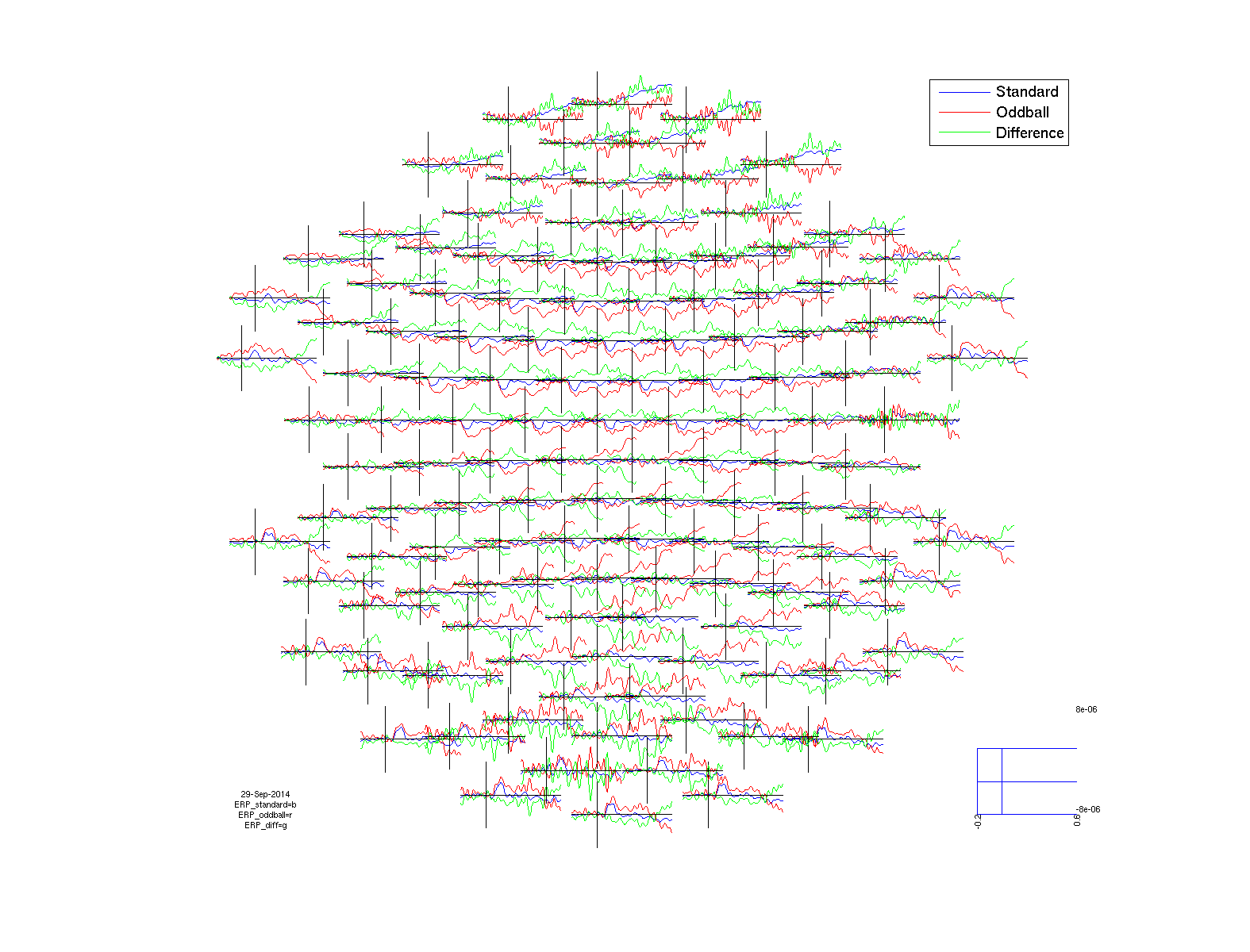Figure: The event-related potentials plotted using ft_multiplotER. The event-related potentials were calculated using ft_preprocessing followed by ft_timelockanalysis

This plots the event-related fields for all sensors arranged topographically according to their position in the helmet. You can use the zoom button (magnifying glass) to enlarge parts of the figure.

Using ft_singleplotER we are going to plot a single EEG channel, for instance ‘EEG020

``````cfg            = [];
cfg.showlabels = 'yes';
cfg.fontsize   = 6;
cfg.layout     = 'natmeg_customized_eeg1005.lay';
cfg.xlim       = [-0.2 0.6];
cfg.ylim       = [-8e-6 8e-6];
cfg.channel    = 'EEG020';

figure;
ft_singleplotER(cfg, ERP_standard, ERP_oddball, ERP_diff);
legend({'Standard';'Oddball';'Difference'});

print -dpng natmeg_erp2.png
``````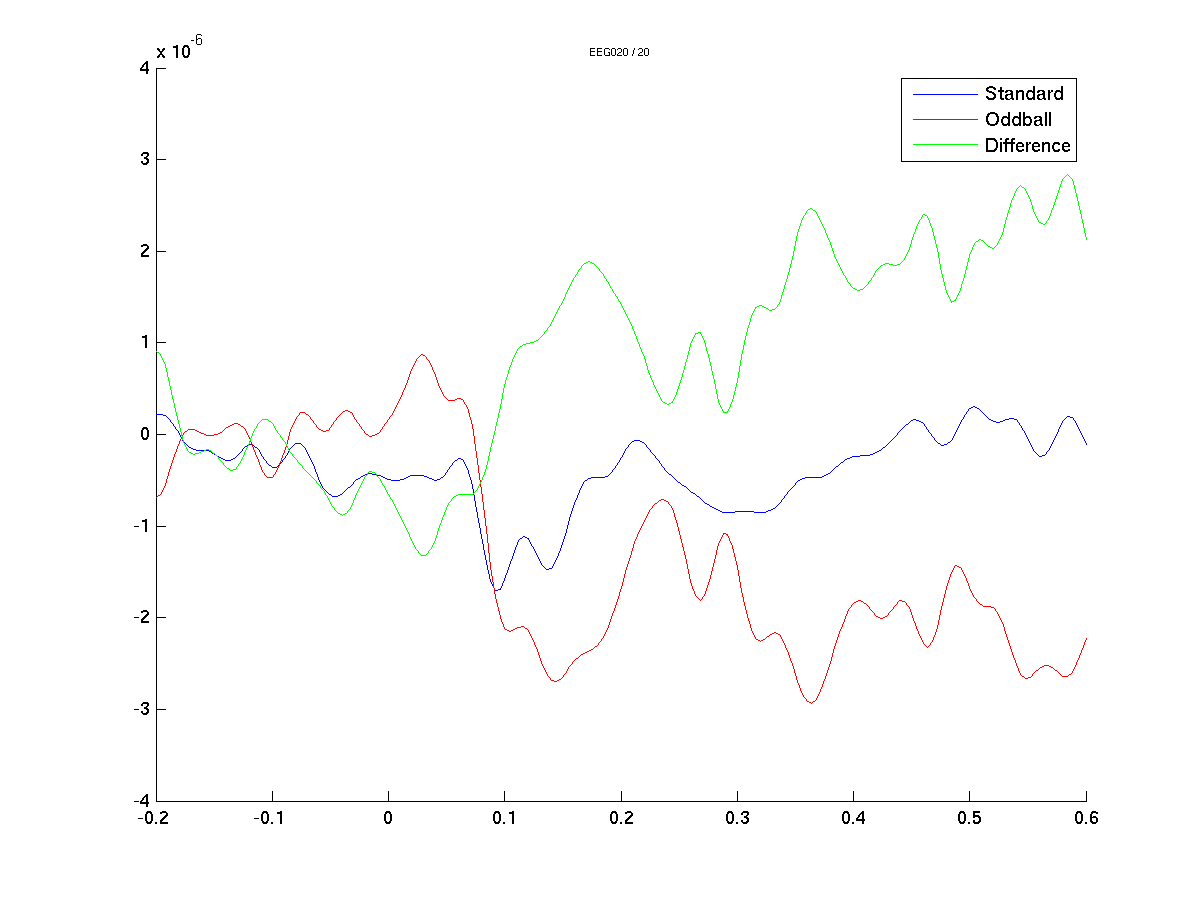Figure: The event-related potentials plotted for three conditions for channel EEG020 using ft_singleplotERFigure: The event-related fields plotted for three conditions for channel MEG0211 using ft_singleplotER

Compare this plot to the single-channel ERFs obtained from the magnetometer data (see Figure 9). Can you identify similar components?

To plot the topographic distribution of the data averaged over the time interval from 0.08 to 0.15 seconds use to following command

``````% Topo
cfg                 = [];
cfg.layout          = 'natmeg_customized_eeg1005.lay';
cfg.zlim            = [-3e-6 3e-6];
cfg.xlim            = [0.08 0.15];
cfg.style           = 'straight';
cfg.comment         = 'no';
cfg.marker          = 'off';
cfg.colorbar        = 'southoutside';

figure;
subplot(1,3,1);
ft_topoplotER(cfg,ERP_standard);
title('Standard');
axis tight

subplot(1,3,2);
ft_topoplotER(cfg,ERP_oddball);
title('Deviant');
axis tight

subplot(1,3,3);
ft_topoplotER(cfg,ERP_diff);
title('Difference');
axis tight

print -dpng natmeg_erp3.png
``````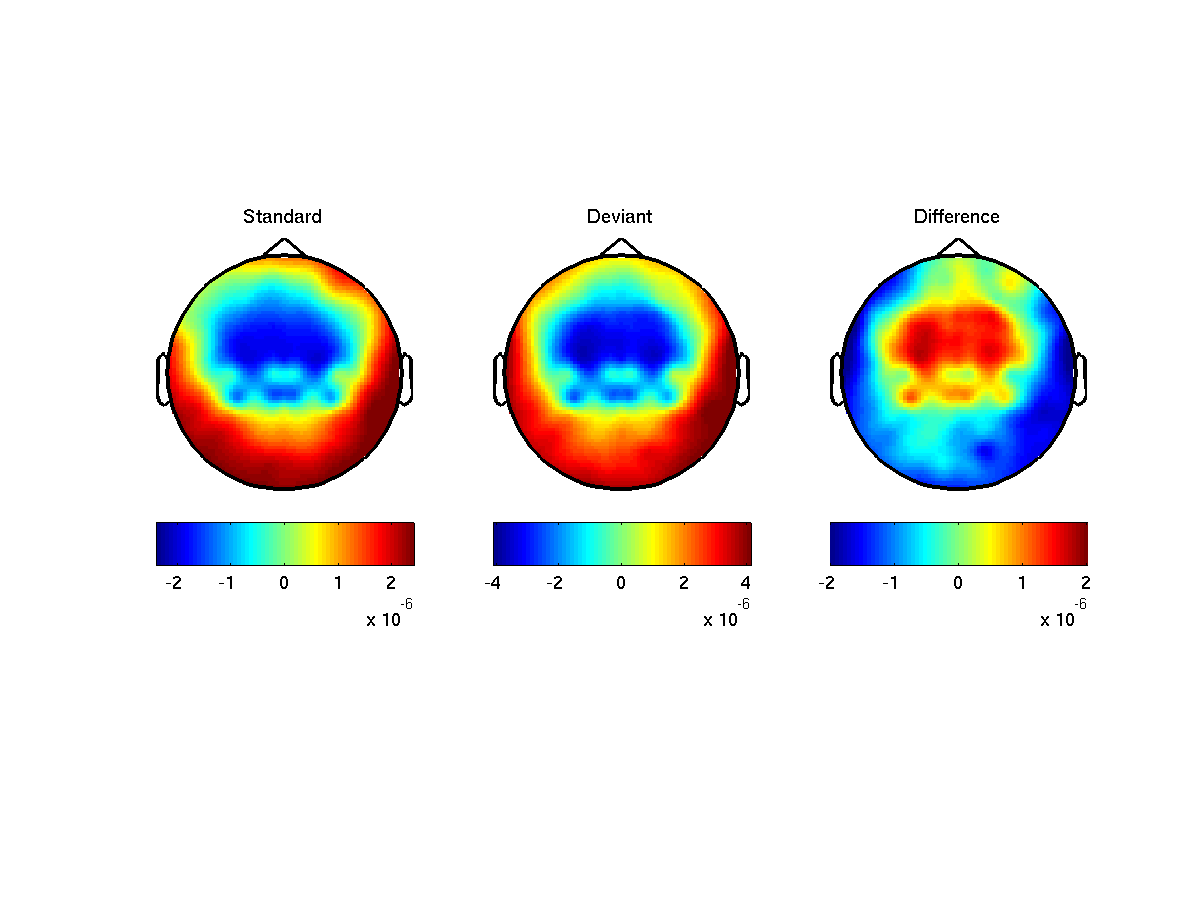Figure: Topographic plot of the event-related potentials obtained using ft_topoplotER

To which MEG channels can we best compare the topographical plots from the EEG data, the magnetometers or the gradiometers?Figure: Topographic plot of the event-related fields (gradiometer) obtained using ft_topoplotER

#### Scalp current density (SCD)

When comparing the EEG topoplots to the MEG topoplots we notice that the spread of the EEG activity measured on the scalp has a wider distribution than when looking at the MEG sensors. Due to volume conduction, most of the focal current generated in the brain spreads over the scalp and it becomes more difficult to localize activity. One method to compensate for this is to calculate scalp current density (SCD), which in its most basic form is the second spatial derivative of the EEG. SCD maps help with localizing the source of activity in the EEG. Furthermore, SCD maps are independent of the choice of reference electrode.

So let’s calculate the SCD on the averaged data.

``````cfg                 = [];
cfg.method          = 'finite';
cfg.elec            = ERP_standard.elec;

scd_ERP_standard    = ft_scalpcurrentdensity(cfg, ERP_standard);
scd_ERP_oddball     = ft_scalpcurrentdensity(cfg, ERP_oddball);
scd_ERP_diff        = ft_scalpcurrentdensity(cfg, ERP_diff);
``````

#### Plotting the results of the SCD

To plot the scalp current density results, use the following code

``````cfg                 = [];
cfg.layout          = 'natmeg_customized_eeg1005.lay'; % name will change
cfg.zlim            = 'maxabs';
cfg.xlim            = [0.08 0.15];
cfg.style           = 'straight';
cfg.comment         = 'no';
cfg.marker          = 'off';
cfg.colorbar        = 'southoutside';

figure;
subplot(1,3,1);
ft_topoplotER(cfg,scd_ERP_standard);
title('Standard');
axis tight;

subplot(1,3,2);
ft_topoplotER(cfg,scd_ERP_oddball);
title('Oddball');
axis tight;

subplot(1,3,3);
ft_topoplotER(cfg,scd_ERP_diff);
title('Difference');
axis tight;

print -dpng natmeg_scd1.png
``````

Note that if you get plotting artifacts in these figures, such as colorbars that do not show completely, you can have a look at this frequently asked question.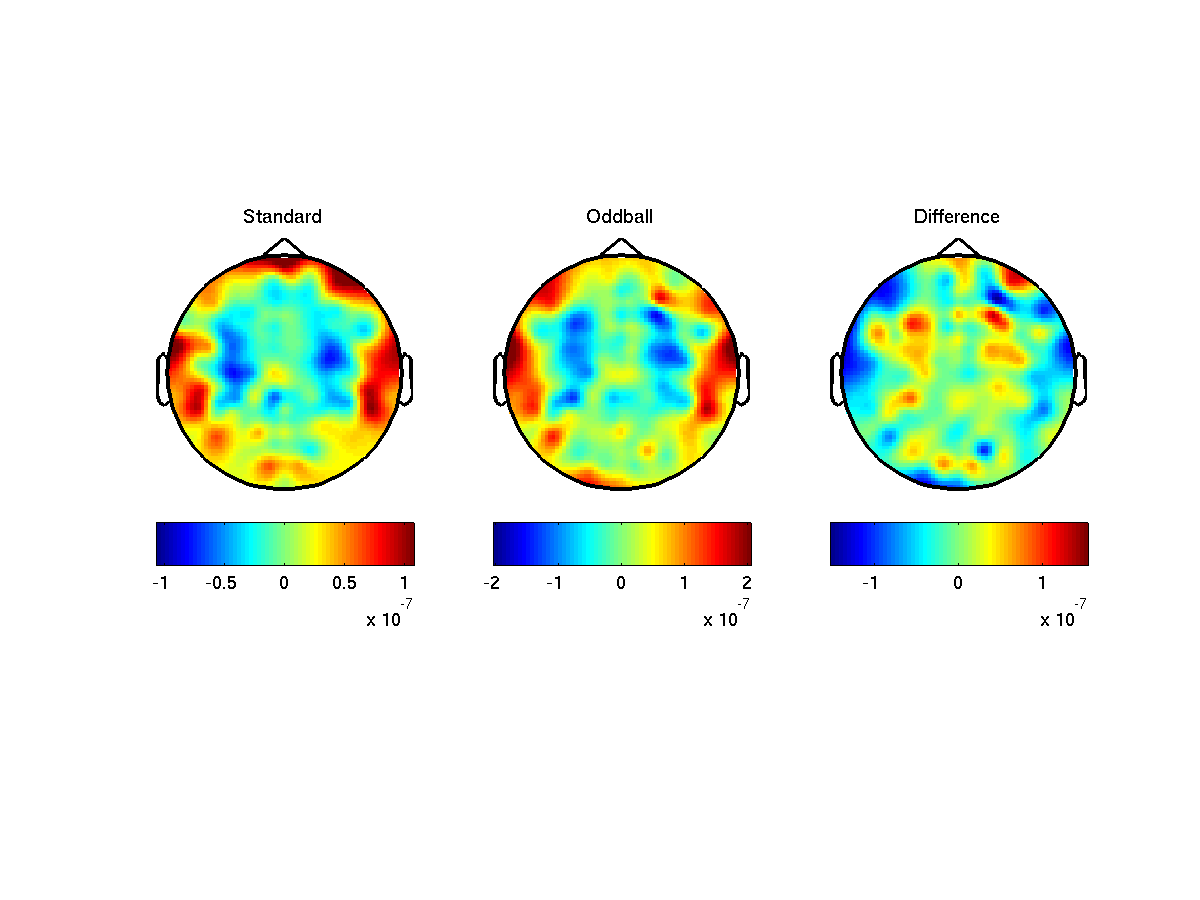Figure: Topoplot of the scalp current density averaged between 0.08 and 0.15 seconds using ft_topoplotER

Did calculating the scalp current density help in narrowing down the source of the EEG activity?

How do these results compare to the MEG results?

## Combining MEG and EEG

So far we have been splitting our combined dataset into separate EEG and MEG datasets. At some point it can be useful to recombine both data subsets into one combined dataset. We can do this using ft_appenddata.

First we will load our data subset

``````load data_EEG
``````

Now we will combine both subsets into a single dataset

``````cfg      = [];
data_all = ft_appenddata(cfg, data_MEG, data_EEG);
``````

As we can see, the new dataset contains all 434 channels (128 EEG + 306 MEG) again

``````data_all =

label: {434x1 cell}
trial: {1x600 cell}
time: {1x600 cell}
sampleinfo: [600x2 double]
trialinfo: [600x3 double]
elec: [1x1 struct]
fsample: 250
cfg: [1x1 struct]
``````

Early on we used ft_rejectvisual to reject trials for the EEG and MEG data separately. The consequence of this is that it is likely that we rejected different trials in the EEG and in the MEG data subset. To avoid this we can run ft_rejectvisual on the complete dataset while still only using a subset of channels for visualization. We can iteratively clean the dataset while looking at a separate subset of channels on each iteration.

First we will clean the dataset based on the EEG channel

``````cfg = [];
cfg.channel    = 'EEG';
cfg.metric     = 'zvalue';
cfg.keepchannel= 'yes';
cfg.layout     = 'neuromag306all.lay';
data_all_clean = ft_rejectvisual(cfg, data_all);
``````

Note that the MEG channels are still in the data. We will now clean the result of the previous operation by looking at the magnetometer channel

``````cfg.channel    = 'MEGMAG';
data_all_clean = ft_rejectvisual(cfg, data_all_clean);
``````

Finally, we will clean the data based on the planar gradiometer

``````cfg.channel    = 'MEGGRAD';
data_all_clean = ft_rejectvisual(cfg, data_all_clean);
``````

We now have the same amount of trials for each type of sensor.

## Summary and suggested further reading

In this tutorial we learned how to look at raw MEG and EEG data, define trials based on trigger codes, preprocess the data - including filtering and re-referencing, and average the data to ERPs and ERFs. We then learned how to display the results in terms of their time courses as well as their corresponding topographies. We also got a good sense of the differences in topographies of fields and potentials when we compared MEG magnetometers with gradiometers and EEG. Finally, we also showed you how you are able to combine EEG and MEG if you would like to do analysis on them simultaneously.

If you are interested in a different analysis of your data that shows event-related changes in the oscillatory components of the signal, you can continue with the combined EEG-MEG timefrequency tutorial or the standard time-frequency analysis tutorial.